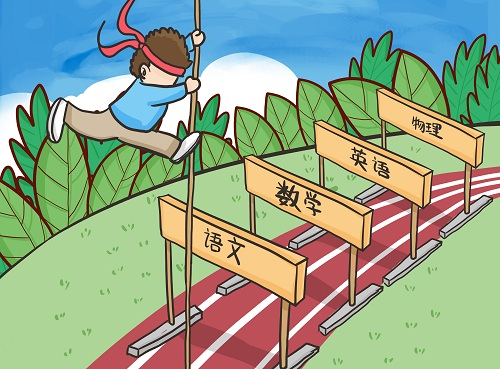# 陕西省级普通高中示范学校名单一览表

截至2017年6月，陕西省普通高中示范性学校共计73所。名单如下：

西安市(30所)

陕西师范大学附属中学(2008.12.17)

陕西省西安中学 (2008.12.17)

西安高新第一中学(2008.12.17)

西安市第八十三中学(2008.12.17)

西北工业大学附属中学(2008.12.17)

西安市铁一中学 (2008.12.17)

西安交通大学附属中学(2008.12.17)

西安市第一中学(2011.6.30)

西北大学附属中学(2011.6.30)

西安市长安区第一中学(2011.6.30)

西安市第八十五中学(2012.6.28)

西安市第八十九中学(2012.6.28)

户县第一中学(2012.6.28)

西安市第七十中学(2013.7.15)

西安市田家炳中学(2013.7.15)

周至中学(2013.7.15)

西安市西光中学(2015.11.6)

西安市第三中学(2015.11.6)

西安市远东第一中学(2015.11.6)

西安市曲江第一中学(2015.11.6)

西安高级中学(2015.11.6)

临潼区华清中学(2015.11.6)

高陵县第一中学(2015.11.6)

阎良区西飞第一中学(2015.11.6)

长安区第二中学(2015.11.6)

西安市航天中学(2017.6.30)

西安市第66中学(2017.6.30)

西安市育才中学(2017.6.30)

西安市第26中学(2017.6.30)

西安市第75中学(2017.6.30)

宝鸡市(7所)

宝鸡市金台高级中学(2015.11.6)

宝鸡中学(2010.9.10)

凤翔中学(2010.12.31)

眉县槐芽中学(2011.12.15)

扶风县扶风高中(2011.12.15)

陈仓区虢镇中学(2013.7.15)

眉县中学(2013.7.15)

咸阳市(9所)

咸阳市实验中学(2010.12.31)

三原南郊中学(2010.12.31)

彩虹中学(2010.12.31)

兴平西郊中学(2010.12.31)

泾阳县泾干中学(2014.2.24)

礼泉县第一中学 (2014.2.24)

武功县普集高级中学(2014.2.24)

陕西咸阳中学(2016.8.31)

咸阳市乾县第一中学(2016.8.31)

铜川市(1所)

铜川市第一中学(2012.6.28)

渭南市(9所)

瑞泉中学(2010.12.31)

象山中学(2010.12.31)

合阳中学(2010.12.31)

渭南高级中学(2011.12.15)

澄城县澄城中学(2011.12.15)

蒲城县尧山中学(2012.6.28)

渭南市杜桥中学(2012.6.28)

白水县白水中学 (2014.9.23)

富平县迤山中学 (2014.9.23)

延安市(1所)

陕西延安中学 (2014.2.24)

榆林市(4所)

陕西省神木中学(2011.12.15)

陕西省榆林中学(2012.6.28)

榆林市第一中学(2015.11.6)

神木县第四中学(2015.11.6)

汉中市(2所)

陕西省汉中中学(2013.7.15)

宁强县天津高级中学(2016.8.31)

安康市(5所)

陕西省安康中学(2011.6.30)

紫阳县紫阳中学(2016.8.31)

安康市白河县高级中学(2016.8.31)

安康市汉阴县中学(2017.6.30)

安康市汉滨区高级中学(2017.6.30)

商洛市(5所)

陕西省商洛中学(2011.6.30)

商南县高级中学(2011.9.30)

陕西省洛南中学(2012.10.31)

陕西省山阳中学(2016.8.31)

陕西省柞水中学 (2017.6.30)

# 热门课程

•### 伊顿名师高一英语听说能力培养一对一辅导课程

•### 伊顿名师人教版高一数学必修1一对一课程辅导

•